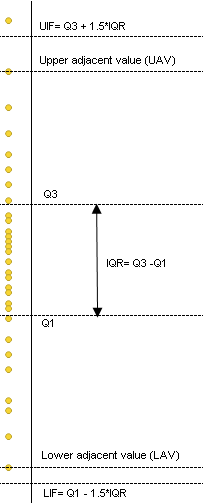Adjacent values Let IQR be the interquartile range. The upper adjacent value (UAV) is the largest observation that is less than or equal to the upper inner fence (UIF), which is the third quartile plus 1.5*IQR. The lower adjacent value (LAV) is the smallest observation that is greater than or equal to the lower inner fence (LIF), which is the first quartile minus 1.5*IQR. Note: If, by the above definition, the UAV is such that it is smaller than Q3, then it is set equal to Q3. Similarly, the LAV is never allowed to be greater than Q1. Outliers Outliers are all values that fall outside either of the fences. Outside values are values that fall in between the inner and outer fences. Far out values are outside the outer fence. The upper outer fence (UOF) is defined as the threshold located at Q3 + (3*IQR). The lower outer fence (LOF )is defined as the threshold located at Q1 – (3*IQR). The outlier percentage is the count of outliers divided by the total count for each category.

Percentiles and Quartiles

Aggregations Overview